# Extract Time or Hour Only from a Date and Time in Excel

This post will guide you how to extract time from a data and time value with a formula in Excel. How do I extract time from a date-time number in Excel 2013/2016. How to extract hour only from the date time format in Excel.

## Extract Time from Date and Time

If you want to extract time from a date and time in Excel, you can use a formula based on the TIME function, The HOUR function, the MINUTE function, and the SECOND function. For example, you want to get time value from a date and time value in Cell B1, you can write down the below formula.

`=TIME(HOUR(B1),MINUTE(B1),SECOND(B1))`

#1 Type this formula into a blank cell C1 and press Enter key.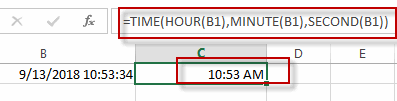#2 Then you need to right click on the Cell C1, and select Format Cell from the pop-up menu list. The Format Cells dialog will open.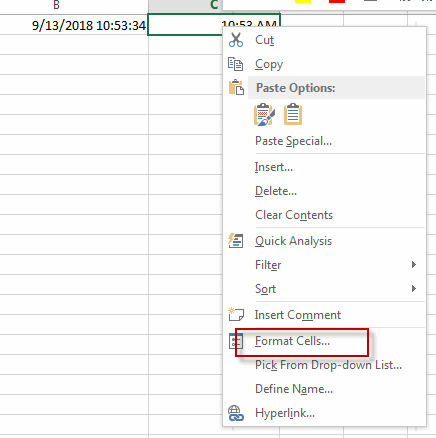#3 switch to Number tab, and click Custom from the Category list. And select one type as: h:mm:ss. Click OK button.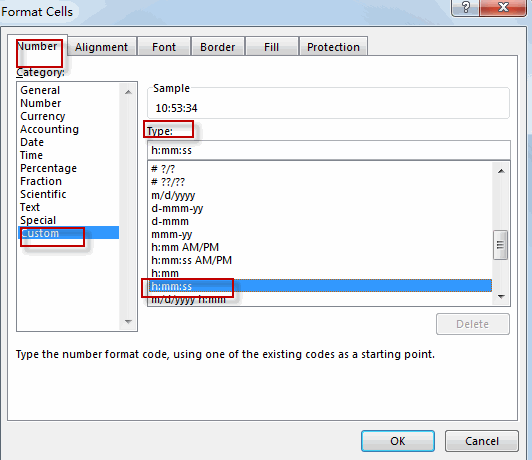#4 let’s see the result.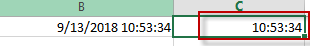## Extract Hour only from Date and Time

If you want to extract hour only, you just need to use the HOUR function. Like this:

`=HOUR(B1)`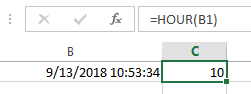If you want to extract minute only from a date and time value, you just need to use the MINUTE function. Like this:

`=MINUTE(B1)`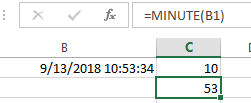If you want to extract second only from a date and time value, you just need to use the SECOND function. Like this:

`=SECOND(B1)`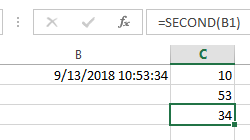### Related Functions

• Excel TIME function
The Excel TIME function returns a date in serial number format for a particular time (the hour, minute and second).The syntax of the TIME function is as below:= TIME(hour, minute, second)…
• Excel HOUR Function
The Excel HOUR function returns the hour of a time value. Or returns an integer value that represent the hour component of a given time. The syntax of the HOUR function is as below:=HOUR (serial_number) …
• Excel MINUTE Function
The Excel MINUTE function returns the minutes of a time value. And the minutes is an integer number from 0 to 59. The syntax of the MINUTE function is as below:=MINUTE (serial_number)…
• Excel SECOND Function
The Excel SECOND function returns the seconds of a time value. Or returns an integer value that represent the second component of a given excel time. And the return value is between 0-59.The syntax of the SECOND function is as below:=SECOND (serial_number)…
Related Posts

Calculate Grades With VLookup in Excel

Why Should You Calculate Grades With VLookup Excel? If you're looking for a simple way to find out a student's grades, VLOOKUP Excel can do the trick. This function uses a lookup table to find the values and sort them ...

Repeating Character n Times in Excel

In daily life, we can use repeated characters to indicate the magnitude or priority of something. For example, a single “!” indicates a minor case, double “!!” indicates a medium case, and triple “!!!” indicates a high case, and so ...

Calculate Cumulative Loan Interest in Excel

What is Cumulative Loan Interest? When comparing different types of loans, many people want to know what is Cumulative Loan Interest. The sum of all interest payments you've made on a loan is referred to as cumulative interest. Different lenders ...

Calculate Cumulative Loan Principal Payments in Excel

How do you Calculate Cumulative Loan Principal Payments in Excel? There are a few different ways to calculate this information, but one way has been proven to be accurate more often than others. One option is to use the CUMPRINC ...

Trap Error or Replace Error by Specific Value with IFERROR function

We often use Excel formulas in our work life, and we may encounter this situation that the formula throws an error and finally an error like #DIV/0! Is displayed in the cell. In today’s tutorial, we will introduce you the ...

Sidebar